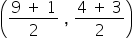Mathematics
Easy

Question

# Find the midpoints for the side of a triangle with vertices (9 , 4) (1 , 3).

## (5 , 4)(5 , 3.5)(7 , 4)(7 , 10)Hint:

## The correct answer is: (5 , 3.5)

### Step 1 of 1:We have to Find the midpoints for the side of a triangle with vertices (9 , 4) (1 , 3)M== (5 , 3.5)

Linear equation in one variable can be solved using fundamental operations

### Related Questions to study#### With Turito Foundation.#### Get an Expert Advice From Turito.